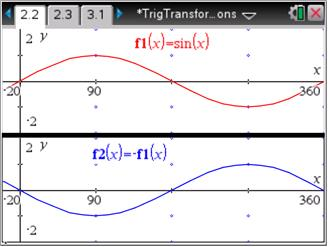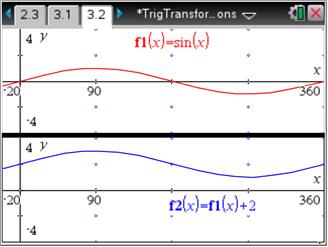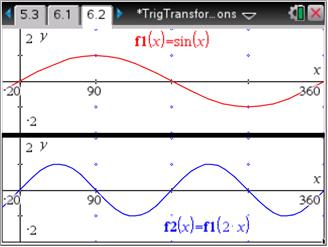# Activities

••• ##### Subject Area

• Math: Algebra II: Transformations
• Math: Algebra II: Trigonometric Functions

• ##### Author9-12

45 Minutes

• ##### Device
•TI-Nspire™ CX/CX II
•TI-Nspire™ CX CAS/CX II CAS
• TI-Nspire™
• TI-Nspire™ CAS
• ##### Software

TI-Nspire™
TI-Nspire™ CAS

3.2

By Pat Flynn

## Trig Transformations

#### Activity Overview

Students will explore function notation and transformational graphing of trigonometric functions.

#### Key Steps

•In transformational graphing part 1, students will observe that multiplying the function by a negative value, results in the graph reflecting over the x-axis.

Students may decide to investigate f(–x). They will observe that the graph is reflected over the y-axis. This points out an interesting fact. With function notation, any changes inside the parentheses usually results in transformations in a horizontal direction, while changes outside the parentheses usually results in transformations in a vertical direction.

•For this problem, the students will discover that adding or subtracting a value to the function will result in vertical translations.

•For this problem, the students will discover that multiplying the variables of a function written in function notation results in a horizontal stretch or compression. To determine the factor by which the function is stretched or compressed, the students need to ask themselves, “What value of x results in a value of 1 for the parentheses?” The answer to this question is "the factor to be multiplied by".